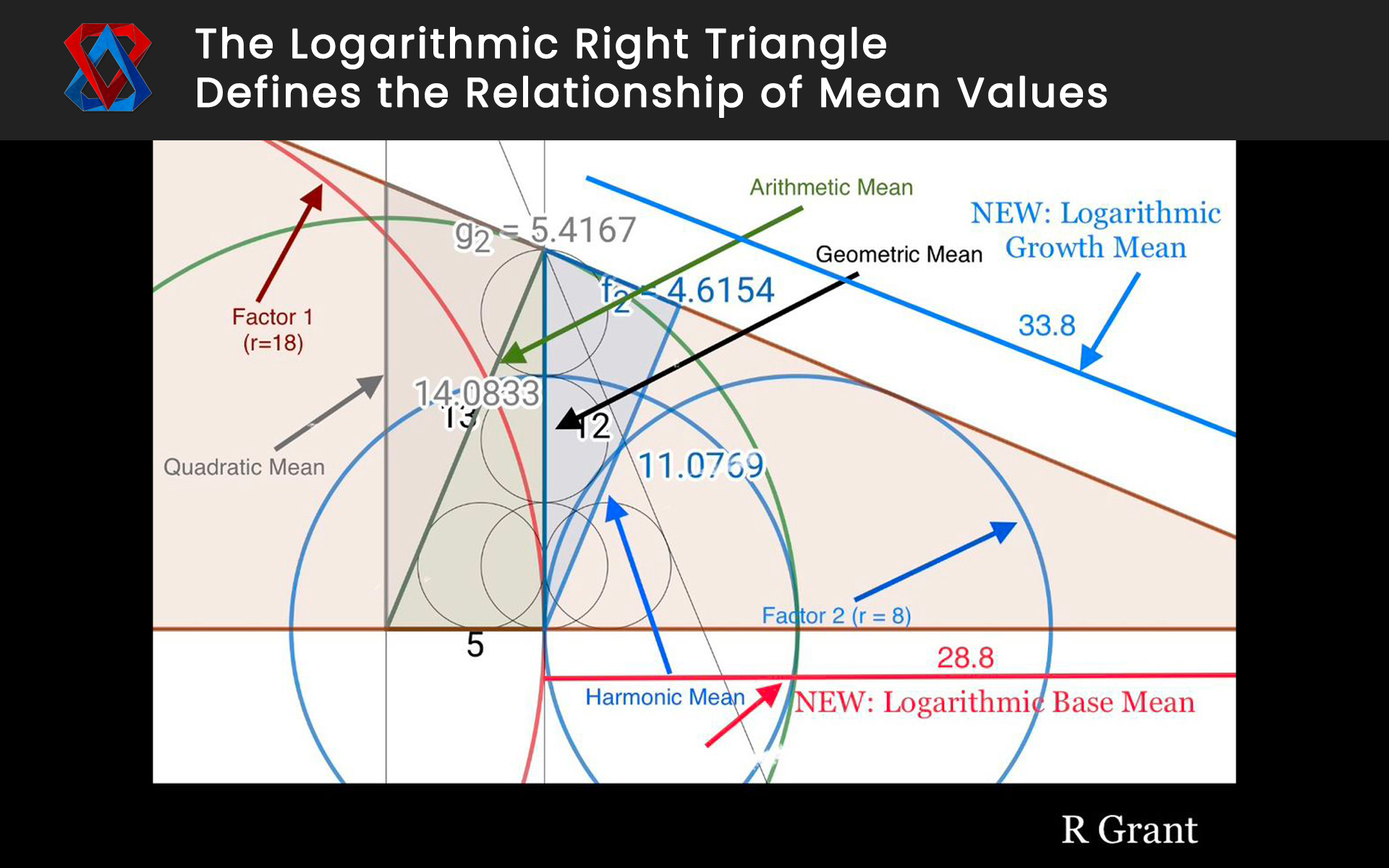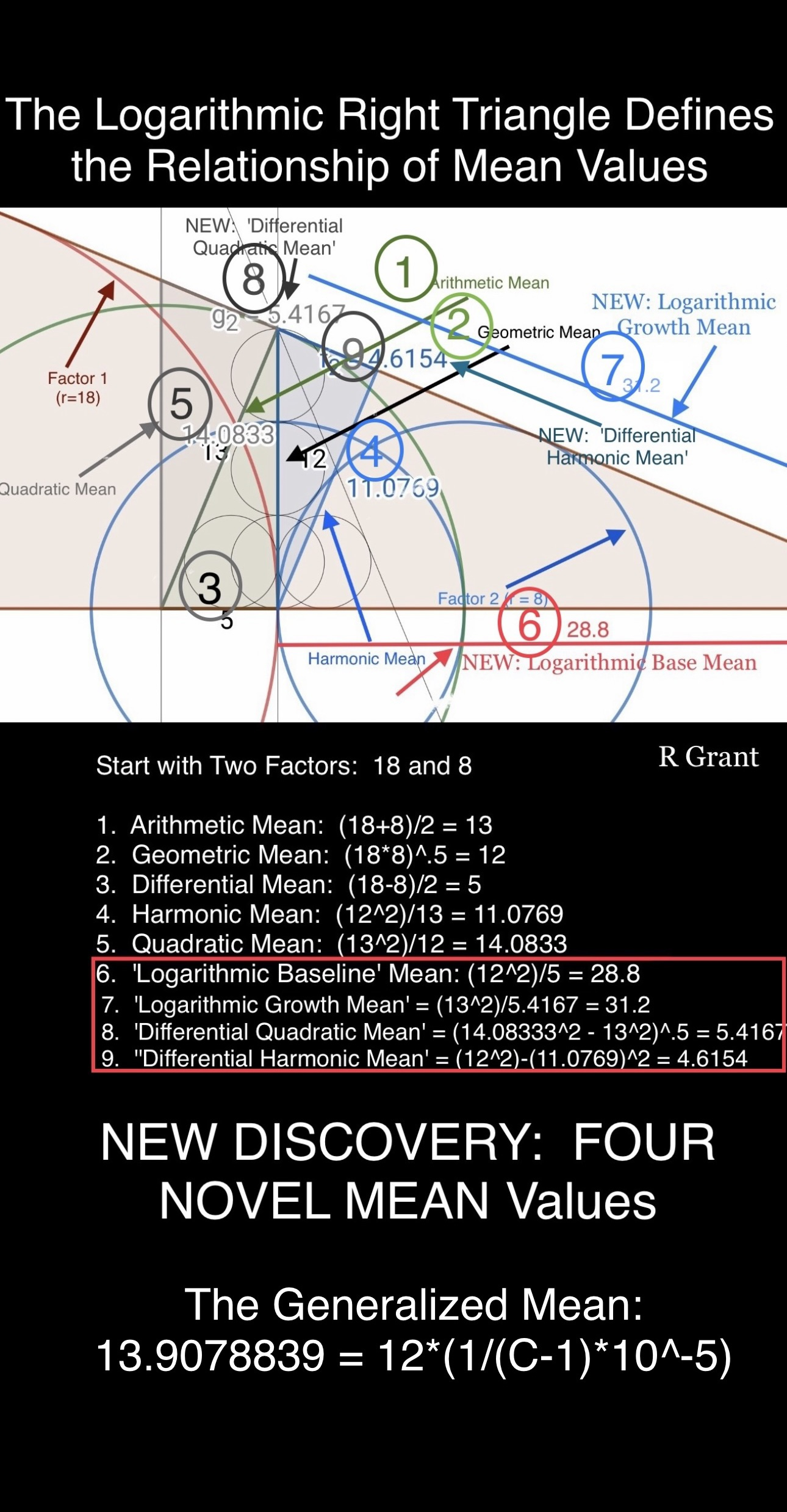### NEW DISCOVERY:

1. The Relationship of the Various Mean Values of Two Factors , namely; 1. Arithmetic 2. Geometric 3. Differential 4. Quadratic and 5. Harmonic are ALL directly derived per the illustration above and are all simply logarithmic expressions of a ‘starting’ Right Triangle. And 2: There are TWO NOVEL MEAN calculations that I have named 1. ‘Logarithmic Baseline Mean’ and 2. ‘Logarithmic Growth Mean’.The above new designations are natural extensions of the aforementioned Mean Values but have not as yet been noted or described in the literature. I believe these NOVEL Mean Values are critical to the understanding of Time as a perceptual construct of the observer.

*NOTE: The above mentioned trigonometric relationships apply to any numerical values### NEW DISCOVERY (updated Sept 22, 2023):

The Logarithmic Right Triangular Relationship that Derives ALL Natural Mean Values including FOUR NOVEL MEAN Values from Two Factors. These relationships exist regardless of the number of input values.

I have named these values the following:

1. ‘Logarithmic Base Mean’
2. ‘Logarithmic Growth Mean’
4. ‘Differential Harmonic Mean’

The relationships are described in the illustration above.

As Background: Pythagoras introduced the concepts of Arithmetic, Geometric, and Harmonic Means in the 6th Century BCE. We do not know whether he was the first to describe such relationships using Right Triangles.

The above NOVEL Discovery logically extends further upon his (Pythagoras’) work to include additional and related Mean Values, which are also derived by symmetrical analysis to include all three side analyses of right triangular associations.

One additional observation: The ‘Generalized Mean’ (defined as the Arithmetic Mean of all nine (9) Associated Mean Values) reveals a possible relationship to the Speed of Light (‘C’) and therefore Time (Time is believed to be associated with the Speed of Light) via the above illustration.